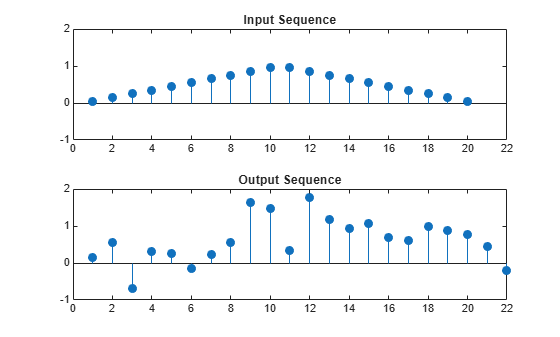# Cross-Correlation of Delayed Signal in Noise

This example shows how to use the cross-correlation sequence to detect the time delay in a noise-corrupted sequence. The output sequence is a delayed version of the input sequence with additive white Gaussian noise. Create two sequences. One sequence is a delayed version of the other. The delay is 3 samples. Add $N\left(0,0.{3}^{2}\right)$ white noise to the delayed signal. Use the sample cross-correlation sequence to detect the lag.

Create and plot the signals. Set the random number generator to the default settings for reproducible results.

```rng default x = triang(20); y = [zeros(3,1);x]+0.3*randn(length(x)+3,1); subplot(2,1,1) stem(x,'filled') axis([0 22 -1 2]) title('Input Sequence') subplot(2,1,2) stem(y,'filled') axis([0 22 -1 2]) title('Output Sequence')```Obtain the sample cross-correlation sequence and use the maximum absolute value to estimate the lag. Plot the sample cross-correlation sequence. The maximum cross correlation sequence value occurs at lag 3, as expected.

```[xc,lags] = xcorr(y,x); [~,I] = max(abs(xc)); figure stem(lags,xc,'filled') hold on stem(lags(I),xc(I),'filled') hold off legend(["Cross-correlation",sprintf('Maximum at lag %d',lags(I))])```Confirm the result using the `finddelay` function.

`finddelay(x,y)`
```ans = 3 ```